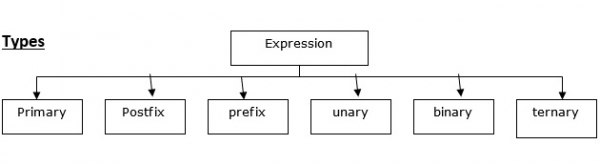# What are types of expressions evaluated in C Language?

An expression is a combination of operators and operands.

Operand is a data item in which operation is performed.

An operator performs an operation on data

For example; z = 3+2*1

z = 5## Types of expressions

The different types of expressions that are evaluated in C language are as follows −

• Primary expressions − The operand in this expression can be a name, a constant or any parenthesized expression. For example, c = a+ (5*b);

• Postfix expressions − In a postfix expression, the operator will be after the operands. For example, ab+

• Prefix expressions − In a prefix expression, the operator is before the operand. For example, +ab

• Unary expression − It contains one operator and one operand. For example, a++, --b

• Binary expression − It contains 2 operands and one operator. For example, a+b, c-d

• Ternary expression − It contains 3 operands and one operator. For example; Exp1? Exp2: Exp3. If Exp1 is true, Exp2 is executed. Otherwise, Exp3 is executed.

## Example

Following is the C program for the expressions which are evaluated in C language −

Live Demo

#include <stdio.h>
main(){
int a , b;
a = 10;
printf( "Value of b is %d", (a == 1) ? 100: 200 );//ternary expression
printf( "Value of b is %d", (a == 10) ? 10: 20 );//ternary expression
}

## Output

When the above program is executed, it produces the following result −

Value of b is 200
Value of b is 10